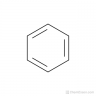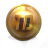#### blackbeard12

##### New member
Hey guys, I encourage you to hop on trading view and throw up "Rex Indicator," very powerful exit indicator that I'm sure some of y'all would like, if anyone knows how to convert it, the code is below. Def worth checking out, beautiful especially with range bars.

Code:
``````//@version=4
study("REX", overlay=false)
// Best used as an exit indicator at default settings.
// Also doing well in entering positions by using TEMA(14) for REX and SMA(14) as signal.
// You need to tune it yourself according to your trading style.
// Use at your own risk.
//
// Vitelot/Yanez/Vts Oct 2019
//
// Oct 2019: added zero line and OS/OB lines (currently commented out)
//
rex_ma_type = input(type=input.string, defval="SMA", options=["EMA","SMA", "Tenkan","TEMA"], title="REX MA type")
smoothing = input(14, title="Smoothing length", minval=1)

sig_ma_type = input(type=input.string, defval="SMA", options=["EMA","SMA", "Tenkan","TEMA"], title="Signal MA type")
smoothing_sig = input(14, title="Signal smoothing length", minval=1)

tenkan(sig, len) =>
0.5 * (highest(sig, len) + lowest(sig, len))

// triple ema
tema(src, len) =>
v2 = ema(src, len)
v = 3 * (v2 - ema(v2, len)) + ema(ema(v2, len), len)  // Triple Exponential
v

ma(t, sig, len) =>
sss = float(na)
if t == "SMA"
sss := sma(sig, len)
if t == "EMA"
sss := ema(sig, len)
if t == "TEMA"
sss := tema(sig, len)
if t == "Tenkan"
sss := tenkan(sig, len)
sss

tvb = 3*close-open-high-low

rex = ma(rex_ma_type, tvb, smoothing)
sig = ma(sig_ma_type, rex, smoothing_sig)

plot(rex, color=#3342c1ff, linewidth=2)
plot(sig, color=#d2a344ff)

hline(0, title="Zero line", color=#ffffff80)

//stddev_period = 200
//bup = 2*stdev(rex,stddev_period)+sma(rex,stddev_period)
//bdn = -2*stdev(rex,stddev_period)+sma(rex,stddev_period)
//plot(bup, color=#ffff0080, style=plot.style_circles, title="Band up")
//plot(bdn, color=#ffff0080, style=plot.style_circles, title="Band down")``````

Last edited by a moderator:
E

#### ezrollin

##### Member
Look at the RSI Divergence indicator instead

•blackbeard12
D

#### diazlaz

##### Well-known member
2019 Donor
VIP
Hi @blackbeard12, take it for a spin, let us know what your thoughts are and how you're currently using it.Ruby:
``````#REX Indicator System V1.0 for ThinkOrSwim
#
#VERSION
# 2020.05.03 @diazlaz - initial port/interpretation.
#
#
#INSTRUCTION
#Best used as an exit indicator at default settings.
#Also doing well in entering positions by using TEMA(14)
#for REX and SMA(14) as signal.
#
#
#CREDITS
#Vitelot/Yanez/Vts Oct 2019
#

declare lower;

input rex_ma_type = {"EMA", default "SMA", "Tenkan", "TEMA"};
input sig_ma_type = {"EMA", default "SMA", "Tenkan", "TEMA"};
input smoothing = 14;
input smoothing_sig = 14;

#def tvb = 3*close-open-high-low;
def tvb = 3*close - (open+high+low);

def rex;
switch (rex_ma_type) {
case "EMA":
rex = ExpAverage(tvb,smoothing);
case "SMA":
rex = Average(tvb,smoothing);
case "Tenkan":
rex = 0.5 * (highest(tvb, smoothing) + lowest(tvb, smoothing));
case "TEMA":
rex = 3 * (ExpAverage(tvb,smoothing) - ExpAverage(ExpAverage(tvb,smoothing), smoothing)) + ExpAverage(ExpAverage(ExpAverage(tvb,smoothing), smoothing), smoothing);
}

def sig;
switch (sig_ma_type) {
case "EMA":
sig = ExpAverage(rex,smoothing_sig);
case "SMA":
sig = Average(rex,smoothing_sig);
case "Tenkan":
sig = 0.5 * (highest(rex, smoothing_sig) + lowest(rex, smoothing_sig));
case "TEMA":
sig = 3 * (ExpAverage(rex,smoothing_sig) - ExpAverage(ExpAverage(rex,smoothing_sig), smoothing_sig)) + ExpAverage(ExpAverage(ExpAverage(tvb,smoothing_sig), smoothing_sig), smoothing_sig);
}

plot pRex = rex;
pRex.SetLineWeight(2);

plot pSig = sig;
plot pZeroline = 0;

#END of REX for ThinkOrSwim 1.0``````

H

#### HighBredCloud

##### Well-known member
VIP
Look at the RSI Divergence indicator instead
Do you have the script for the RSI Divergence? Is it this one?
Code:
``````# RSI_With_Divergence
# Mobius
# V01.01.2013
# 4.15.2019
#hint:<b>RSI with Divergence</b>

# Note: Install this as a new study. Save this study using the name above (the first line of code RSI_With_Divergence).

# To use this study as a scan; DO NOT TRY TO LOAD IT DIRECTLY IN THE SCANNER, IT WILL THROW AN ERROR MESSAGE. Go to the scan tab. Delete any existing scan criteria. Click Add Study Filter. Click the window under Criteria. In that drop down menu click Custom. Delete the existing study. Click Add Condition. Click the down arrow in the Select A Condition window. Click Study. Scroll down the List till you find RSI_With_Divergence and click it. Click on the Plot window and you can choose Dhigh or Dlow in addition to the default plot RSI. If you choose either of the divergence siganls choose is True from the center column. Click on the aggregation period at the top left and set the aggregation period you want scaned. Then click Save and when the popup window shows the warning that this is a custom scan chose OK. Now put the list of stocks you wish to scan in the Scan In box and chose any list you want that to intersect with. If you wish to make this a Dynamic WatchList, save this scan with a name such as RSI_With_Div_WL then in your Gadgets box click the little gear icon, locate the name of the scan you just saved and click it. As equities match the scan criteria they will populate the list.

declare lower;

input n = 14;        #hint nRSI: Periods or length for RSI

input Over_Bought = 70; #hint Over_Bought: Over Bought line

input Over_Sold = 30;   #hint Over_Sold: Over Sold line

def o = open;

def h = high;

def l = low;

def c = close;

def x = BarNumber();

def MidLine = 50;

def NetChgAvg = ExpAverage(c - c, n);

def TotChgAvg = ExpAverage(AbsValue(c - c), n);

def ChgRatio = if TotChgAvg != 0

then NetChgAvg / TotChgAvg

else 0;

plot RSI = 50 * (ChgRatio + 1);

RSI.AssignValueColor(if RSI < Over_Sold

then color.yellow

else if RSI > Over_Bought

then color.yellow

else createColor(25, 75, 250));

plot OverSold = Over_Sold;

plot OverBought = Over_Bought;

def bar = BarNumber();

def Currh = if RSI > OverBought

then fold i = 1 to Floor(n / 2)

with p = 1

while p

do RSI > getValue(RSI, -i)

else 0;

def CurrPivotH = if (bar > n and

RSI == highest(RSI, Floor(n/2)) and

Currh)

then RSI

else double.NaN;

def Currl = if RSI < OverSold

then fold j = 1 to Floor(n / 2)

with q = 1

while q

do RSI < getValue(RSI, -j)

else 0;

def CurrPivotL = if (bar > n and

RSI == lowest(RSI, Floor(n/2)) and

Currl)

then RSI

else double.NaN;

def CurrPHBar = if !isNaN(CurrPivotH)

then bar

else CurrPHBar;

def CurrPLBar = if !isNaN(CurrPivotL)

then bar

else CurrPLBar;

def PHpoint = if !isNaN(CurrPivotH)

then CurrPivotH

else PHpoint;

def priorPHBar = if PHpoint != PHpoint

then CurrPHBar

else priorPHBar;

def PLpoint = if !isNaN(CurrPivotL)

then CurrPivotL

else PLpoint;

def priorPLBar = if PLpoint != PLpoint

then CurrPLBar

else priorPLBar;

def HighPivots = bar >= highestAll(priorPHBar);

def LowPivots = bar >= highestAll(priorPLBar);

def pivotHigh = if HighPivots

then CurrPivotH

else double.NaN;

plot PlotHline = pivotHigh;

PlotHline.enableApproximation();

PlotHline.SetDefaultColor(GetColor(7));

PlotHline.SetStyle(Curve.Short_DASH);

plot pivotLow = if LowPivots

then CurrPivotL

else double.NaN;

pivotLow.enableApproximation();

pivotLow.SetDefaultColor(GetColor(7));

pivotLow.SetStyle(Curve.Short_DASH);

plot PivotDot = if !isNaN(pivotHigh)

then pivotHigh

else if !isNaN(pivotLow)

then pivotLow

else double.NaN;

pivotDot.SetDefaultColor(GetColor(7));

pivotDot.SetPaintingStrategy(PaintingStrategy.POINTS);

pivotDot.SetLineWeight(3);

# End Code RSI with Divergence``````

K

#### KKaiser

##### Member
VIP
Will test it today.

C

#### CreaitiveFreedom

##### New member
Gave the REX script a quick back test. Lots of options. Thanks for converting this. It's going to take some tweaking if it's going to fit into my system.Custom Take Profit Exit Questions 2